## Learning objectives

• Learn how to create a useful and attractive barplot and boxplot using ggplot.
• Create barplot/boxplot where color and size of the points vary with variables and values.

## Content

The tutorial will guide from beginner level (level 1) to the Pro level in barplot and boxplot. Some of the functions used in this tutorial are introduced in the scatter plot tutorial, Below is the list of topics that are covered in this page.

• Simple barplot using ggplot
• Edit color and format of barplot
• Barplot by group
• Order variables in barplot
• Barplot in multiple panels
• Export ggplot image
• Simple boxplot using ggplot
• Edit format of boxplot
• Boxplot by group
• log10 scale
``````# We will use "iris" dataset that comes in R by default. Check the data
summary(iris)``````
``````##   Sepal.Length    Sepal.Width     Petal.Length    Petal.Width
##  Min.   :4.300   Min.   :2.000   Min.   :1.000   Min.   :0.100
##  1st Qu.:5.100   1st Qu.:2.800   1st Qu.:1.600   1st Qu.:0.300
##  Median :5.800   Median :3.000   Median :4.350   Median :1.300
##  Mean   :5.843   Mean   :3.057   Mean   :3.758   Mean   :1.199
##  3rd Qu.:6.400   3rd Qu.:3.300   3rd Qu.:5.100   3rd Qu.:1.800
##  Max.   :7.900   Max.   :4.400   Max.   :6.900   Max.   :2.500
##        Species
##  setosa    :50
##  versicolor:50
##  virginica :50
##
##
## ``````
``````# Lets say we want to create a barplot of the mean Petal Length under each Species
# Calculating mean for each Species
df = aggregate(iris[,1:4], by = list(iris\$Species), FUN = mean)
df``````
``````##      Group.1 Sepal.Length Sepal.Width Petal.Length Petal.Width
## 1     setosa        5.006       3.428        1.462       0.246
## 2 versicolor        5.936       2.770        4.260       1.326
## 3  virginica        6.588       2.974        5.552       2.026``````
``````# Typical barplot
barplot(Petal.Length~Group.1, data = df,xlab = c('Species'), ylab = c('Petal Length'))``````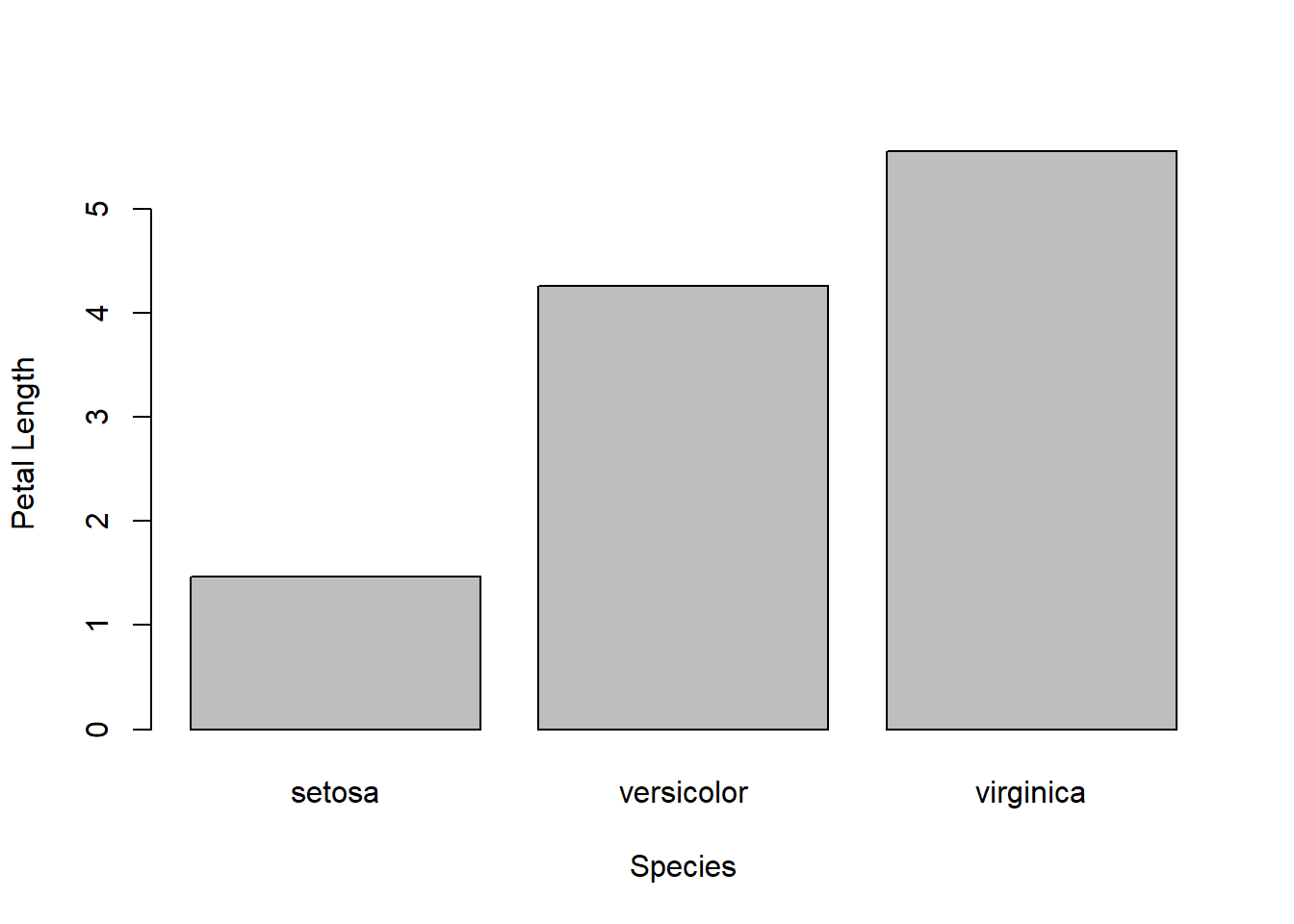## Bar plot using ggplot

### Level 1: Simple ggplot

``library(ggplot2)``
``## Warning: package 'ggplot2' was built under R version 4.0.3``
``````ggplot(df, aes(x = Group.1, y = Petal.Length)) +
geom_bar(stat="identity") +
xlab('Species') + ylab('Petal Length')``````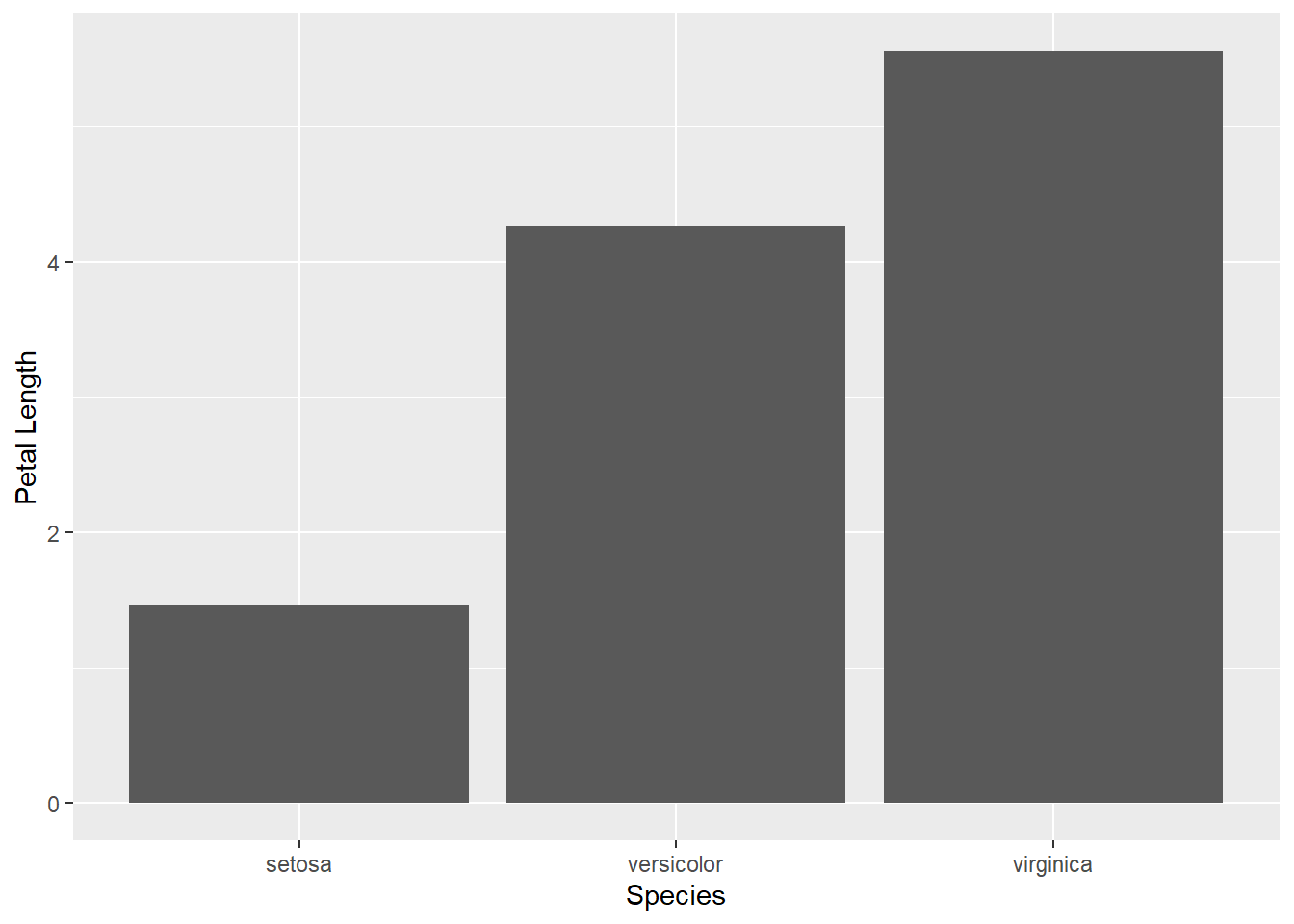### Level 2: Edit color and format

Stepwise tutorial of editing each item is shown in an earlier tutorial on scatter plot Here, the functions are directly applied.

``````p = ggplot(df, aes(x = Group.1, y = Petal.Length)) +
geom_bar(stat="identity",fill="#56B4E9",color='black') +
xlab('Species') + ylab('Petal Length') + theme_bw()+
theme(text = element_text(size=12),
axis.text.x = element_text(angle=0, hjust=.5),
plot.title = element_text(hjust = 0.5),
plot.subtitle = element_text(hjust = 0.5))+
scale_y_continuous(breaks = seq(0, 6, by=1), limits=c(0,6))+
ggtitle("Barplot using ggplot")

p1 = p + geom_hline(yintercept=3.5, linetype="dashed",
color = "red", size=1)+
ggtitle("Barplot with horizontal line")

p2 = p1 + coord_flip()+
ggtitle("Flipped coordinate")

library(gridExtra)
grid.arrange(p, p1, p2, ncol=3)``````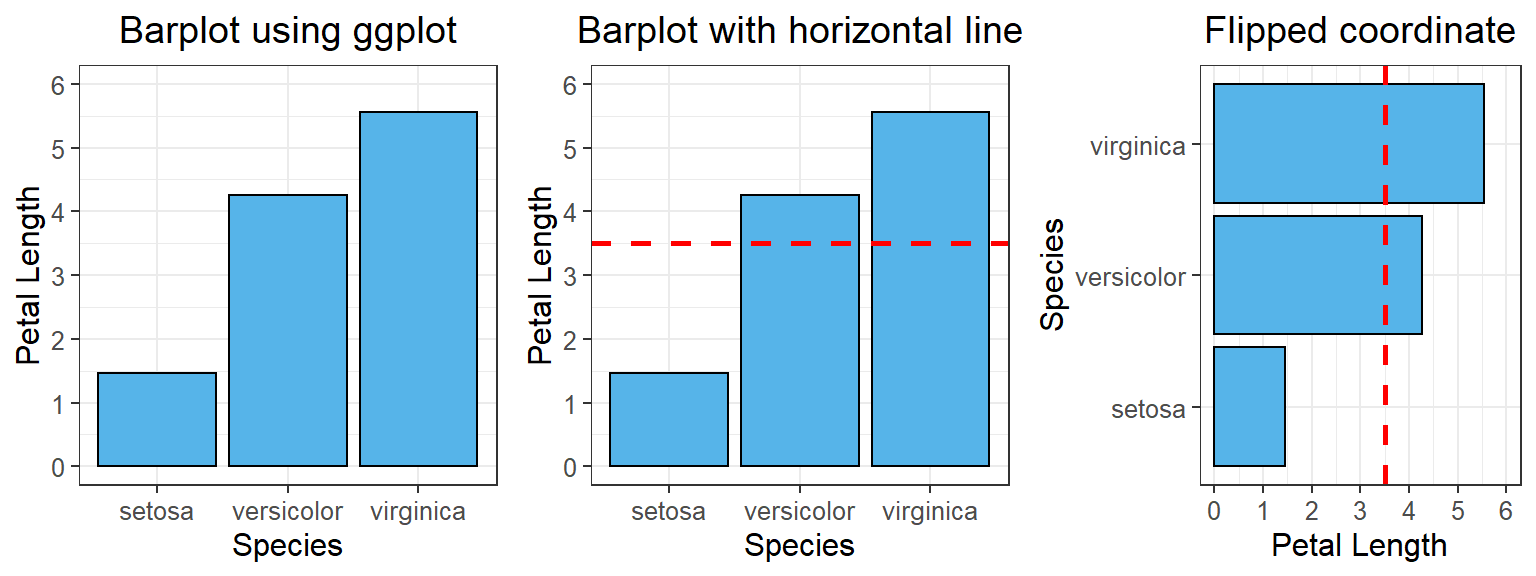### Level 3: Barplot by group

• To plot multiple variables in the same barplot, we need to arrange the data in a suitable format.
• Lets first see how the iris data looks like after rearranging. It would be easier to explain from that.
• We will use a function called melt under the library “reshape2”.
``````library(reshape2) # if not installed, then install using install.packages('reshape2')
md.df = melt(df, id.vars = c('Group.1'))
# md.df has three columns where we brought all variables in same column and all Species info in the same column.
``````##      Group.1     variable value
## 1     setosa Sepal.Length 5.006
## 2 versicolor Sepal.Length 5.936
## 3  virginica Sepal.Length 6.588
## 4     setosa  Sepal.Width 3.428
## 5 versicolor  Sepal.Width 2.770
## 6  virginica  Sepal.Width 2.974``````
``````p = ggplot(md.df, aes(x = Group.1, y = value, group = variable, fill = variable)) +
geom_bar(stat="identity",color='black', position = "dodge") +
xlab('Species') + ylab('Values') + theme_bw()+
theme(text = element_text(size=16),
axis.text.x = element_text(angle=0, hjust=.5),
plot.title = element_text(hjust = 0.5),
plot.subtitle = element_text(hjust = 0.5))+
ggtitle("Barplot by group")

p1 = p + scale_fill_discrete(name = "Characteristics", labels = c("Sepal Length", "Sepal Width", "Petal Length", "Petal Width"))

grid.arrange(p, p1, ncol=2)``````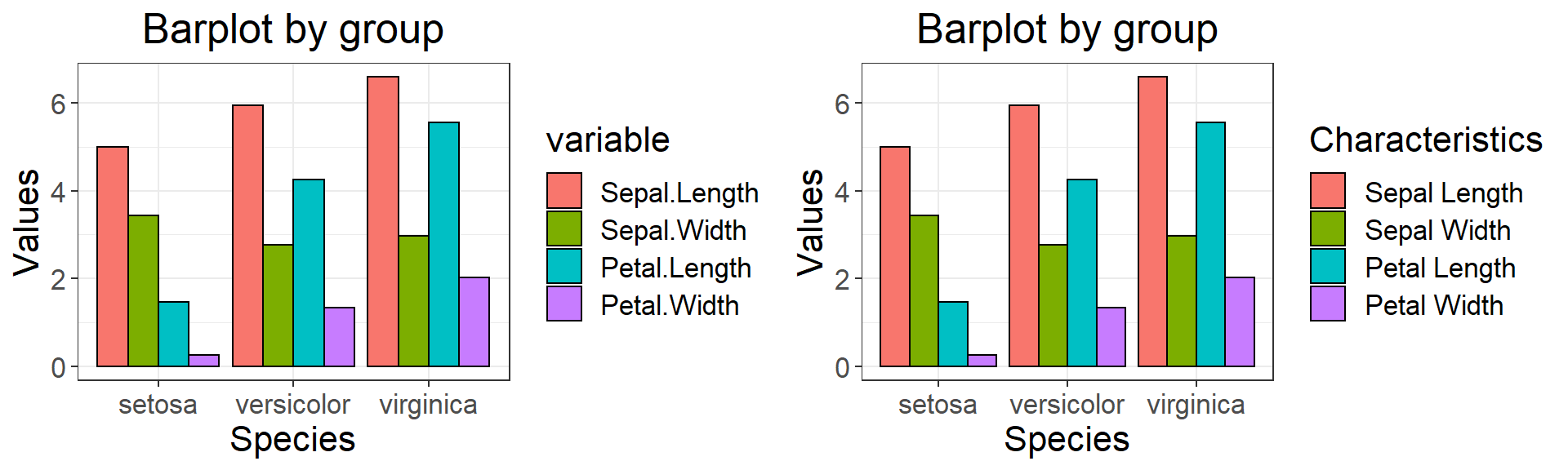### Level 4: Order variables in barplot

• There are multiple ways to order barplot by variables. I found the following method the easiest.
``````# use the following three lines of code to order the plot. Here, tmp stores the desired order
tmp = c("virginica","setosa","versicolor")
md.df2 = md.df[order(match(md.df\$Group.1, tmp)),]
md.df2\$Group.1 = factor(as.character(md.df2\$Group.1), levels = unique(md.df2\$Group.1))

ggplot(md.df2, aes(x = Group.1, y = value, group = variable, fill = variable)) +
geom_bar(stat="identity",color='black', position = "dodge") +
xlab('Species') + ylab('Values') + theme_bw()+
ylim(0,8)+
theme(text = element_text(size=16),
axis.text.x = element_text(angle=0, hjust=.5),
plot.title = element_text(hjust = 0.5),
plot.subtitle = element_text(hjust = 0.5))+
ggtitle("Order variables in barplot")+
geom_text(aes(label=value), vjust=-0.3, size=4, # adding values
position = position_dodge(0.9))``````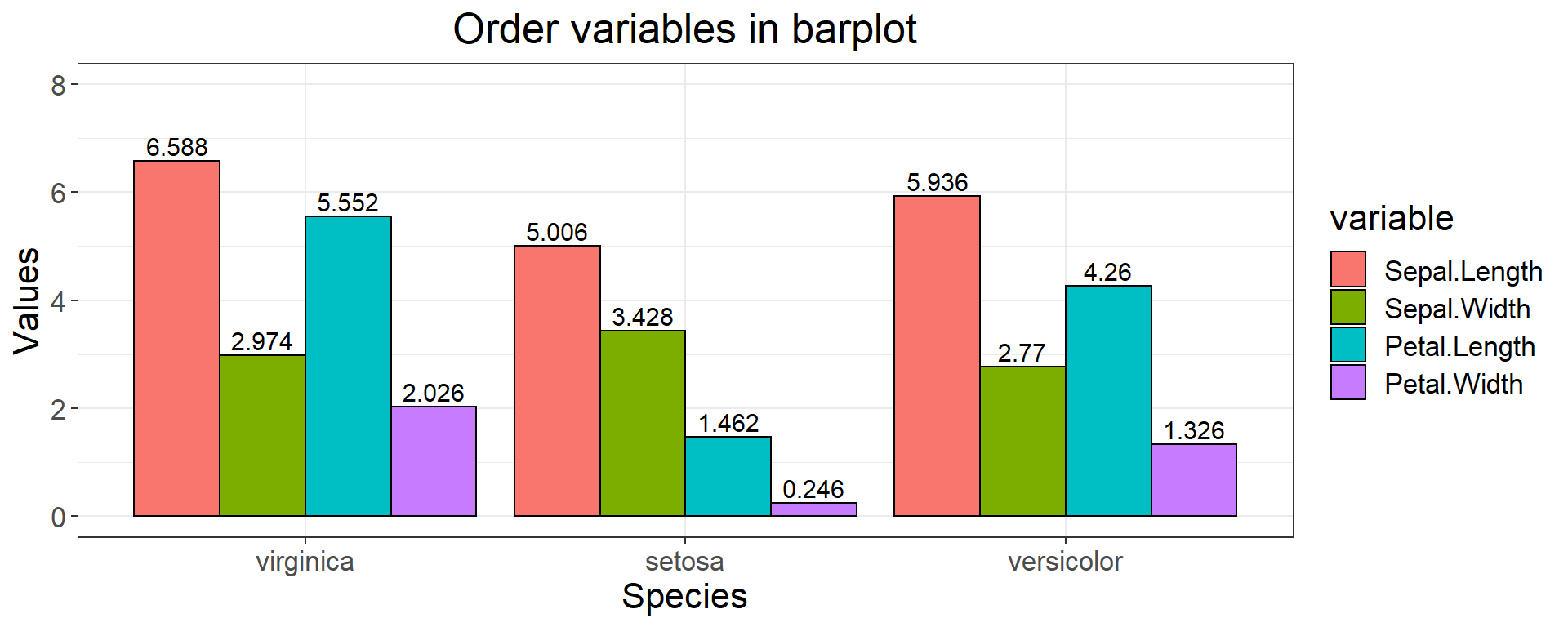### Level 5: Barplot in multiple panels

• Use facet_wrap() function to plot in multiple panels.
``````p = ggplot(md.df, aes(x = Group.1, y = value, fill = variable)) +
geom_bar(stat="identity",color='black', position = "dodge") +
xlab('Species') + ylab('Values') + theme_bw()+
theme(text = element_text(size=16),
axis.text.x = element_text(angle=0, hjust=.5),
plot.title = element_text(hjust = 0.5),
plot.subtitle = element_text(hjust = 0.5),
legend.position = 'none')+ #removing legend
ggtitle("Barplot in multiple panels")+
facet_wrap(~ variable, ncol = 2)

p``````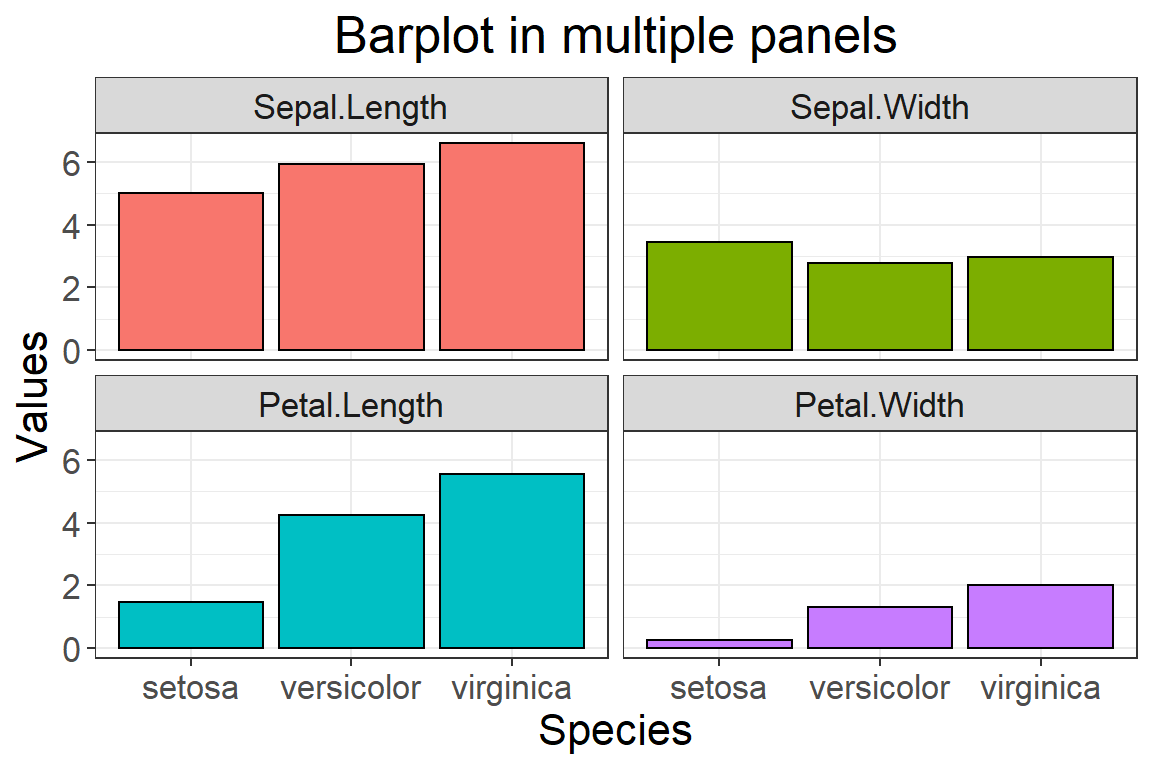### Export ggplot image

``````#setting working directory to export image.
setwd('C:/sarfaraz/Project_R_tutorials/R-tutorial/R_beginner_part3_files/')
ggsave(p,filename="barplot.png",
width = 20, height = 15, units = "cm")``````

## Boxplot using ggplot

``````# Typical boxplot
boxplot(Petal.Length~Species, data = iris, xlab = c('Species'), ylab = c('Petal Length'))``````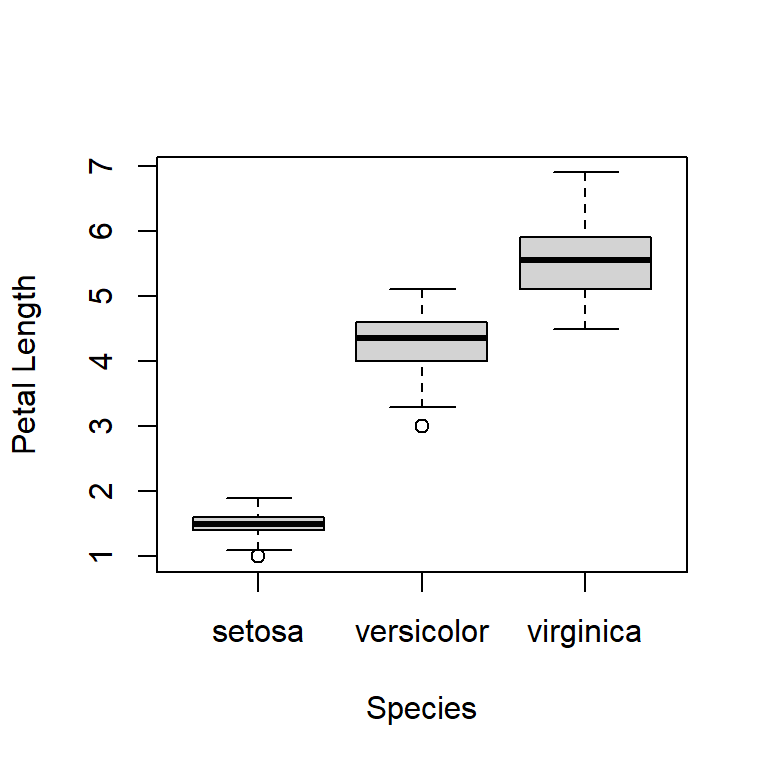### Level 1: Simple ggplot

``````ggplot(iris, aes(x = Species, y = Petal.Length)) +
geom_boxplot() +
xlab('Species') + ylab('Petal Length')``````